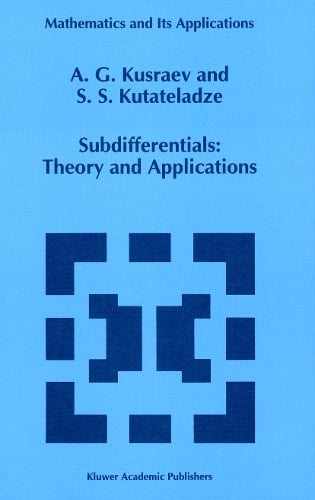•# Subdifferentials: Theory and Applications - Mathematics and Its Applications 323 (Paperback)

(author), (author)
£99.99
Paperback 405 Pages / Published: 13/10/2012
• We can order this

Usually dispatched within 3 weeks

The subject of the present book is sub differential calculus. The main source of this branch of functional analysis is the theory of extremal problems. For a start, we explicate the origin and statement of the principal problems of sub differential calculus. To this end, consider an abstract minimization problem formulated as follows: x E X, f(x) --+ inf. Here X is a vector space and f : X --+ iR is a numeric function taking possibly infinite values. In these circumstances, we are usually interested in the quantity inf f( x), the value of the problem, and in a solution or an optimum plan of the problem (i. e. , such an x that f(x) = inf f(X", if the latter exists. It is a rare occurrence to solve an arbitrary problem explicitly, i. e. to exhibit the value of the problem and one of its solutions. In this respect it becomes necessary to simplify the initial problem by reducing it to somewhat more manageable modifications formulated with the details of the structure of the objective function taken in due account. The conventional hypothesis presumed in attempts at theoretically approaching the reduction sought is as follows. Introducing an auxiliary function 1, one considers the next problem: x EX, f(x) -l(x) --+ inf. Furthermore, the new problem is assumed to be as complicated as the initial prob lem provided that 1 is a linear functional over X, i. e.

Publisher: Springer
ISBN: 9789401041171
Number of pages: 405
Weight: 662 g
Dimensions: 240 x 160 x 22 mm
Edition: Softcover reprint of the original 1st ed. 199